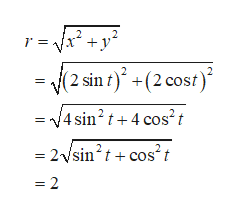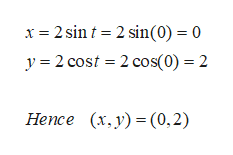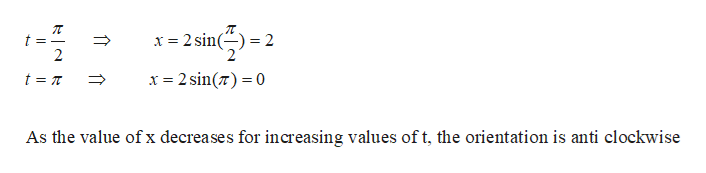# The position of an object in circular motion is modeled by the given parametric equations. Describe the path of the object by stating the radius of the circle, the position at time t = 0, the orientation of the motion (clockwise or counterclockwise), and the time t that it takes to complete one revolution around the circle. x = 2 sin(t), y = 2 cos(t)

Question
47 views

The position of an object in circular motion is modeled by the given parametric equations. Describe the path of the object by stating the radius of the circle, the position at time t = 0, the orientation of the motion (clockwise or counterclockwise), and the time t that it takes to complete one revolution around the circle.
x = 2 sin(t), y = 2 cos(t)

check_circle

Step 1

The two parametric equations are, x=2sint and y=2cost

For calculating the radius of the circle, we use the formula,help_outlineImage Transcriptionclose|x2 +y r = (2 sin t) +(2 cost) =4 sin2 t+4 cos't 2/sin2tcos2t 2 fullscreen
Step 2

Position of the object at a given time t=0 is (0,2)help_outlineImage Transcriptionclosex 2 sin 2 sin(0) = 0 y 2 cost 2 cos(0) 2 Hence (x,y) (0,2) fullscreen
Step 3

Orientation of the object;

Substitute x= π/2 and π and check the corresponding value for x.

• If the value for x decreases for increasing values of t, then t...help_outlineImage Transcriptionclosex 2 sin x 2 sin( 0 As the value of x decreases for increasing values of t, the orientation is anti clockwise fullscreen

### Want to see the full answer?

See Solution

#### Want to see this answer and more?

Solutions are written by subject experts who are available 24/7. Questions are typically answered within 1 hour.*

See Solution
*Response times may vary by subject and question.
Tagged in

### Functions